# Identify p(t), q(t) and r(t). Solve the given initial value problem using the method of Laplace...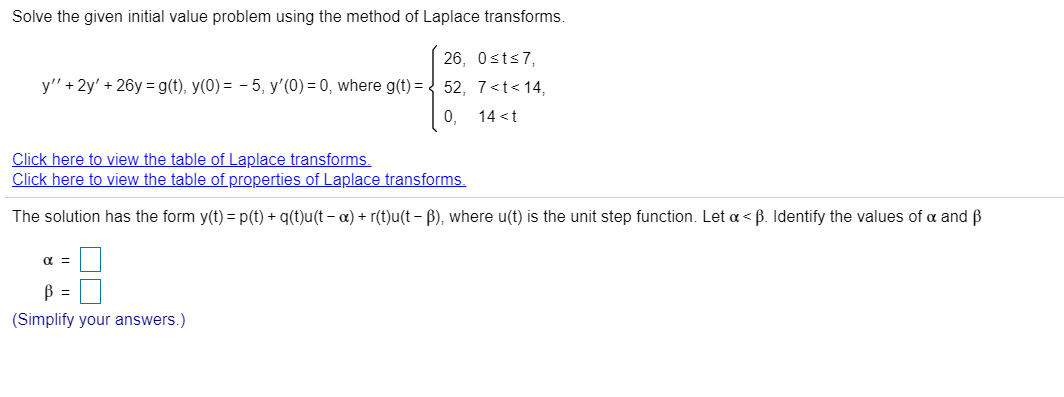Identify p(t), q(t) and r(t).

Solve the given initial value problem using the method of Laplace transforms. 26, Osts 7 y' + 2y' + 20y = g(t), y(0)= -5, y'(O)= 0, where g(t) = { 52, 7<t< 14, 0, 14<t Click here to view the table of Laplace transforms. Click here to view the table of properties of Laplace transforms. The solution has the form y(t) = P(t)+q(t)u(t-a) + r(t)u(t - ), where u(t) is the unit step function. Let a<B. Identify the values of a and B E B B = (Simplify your answers.)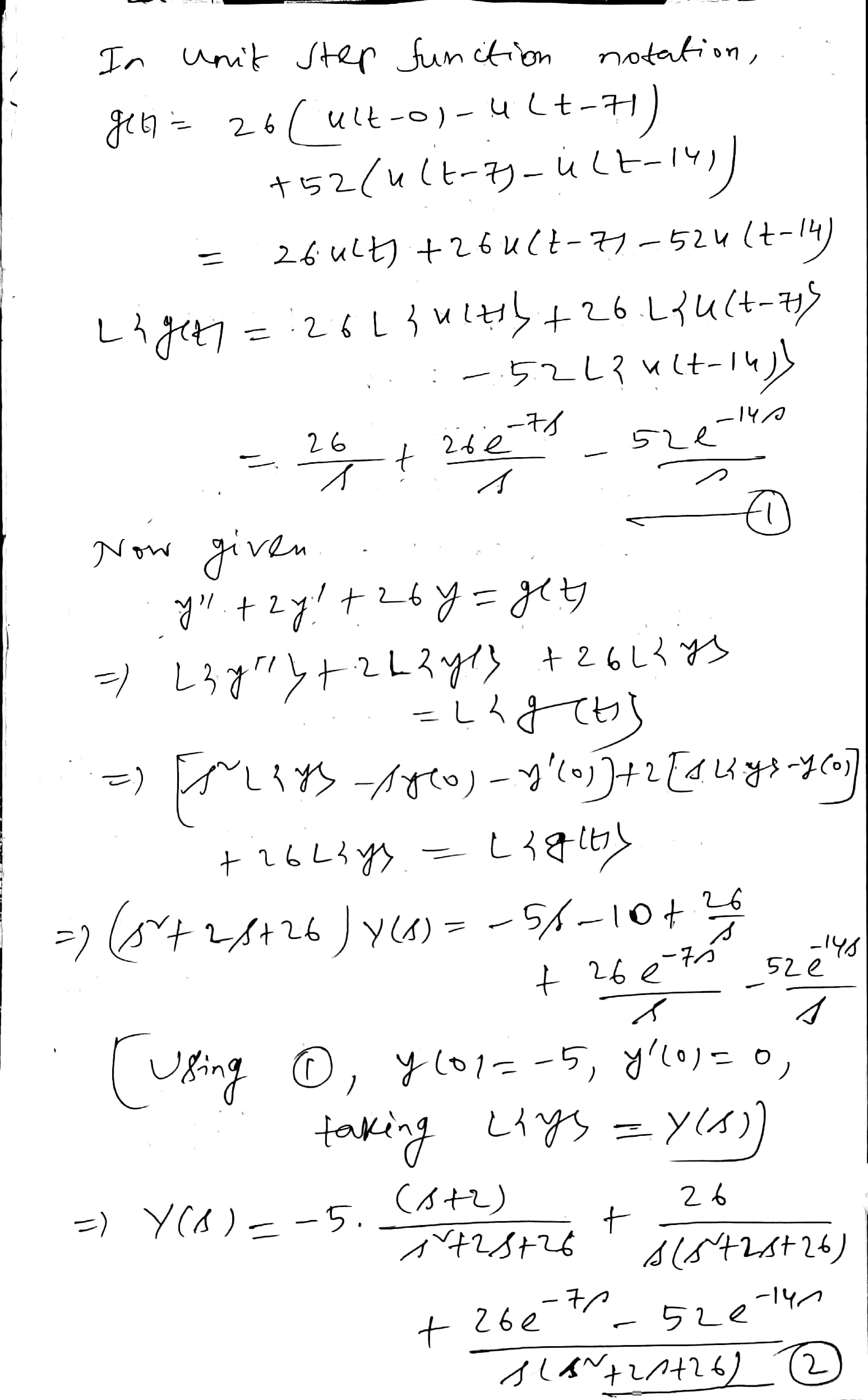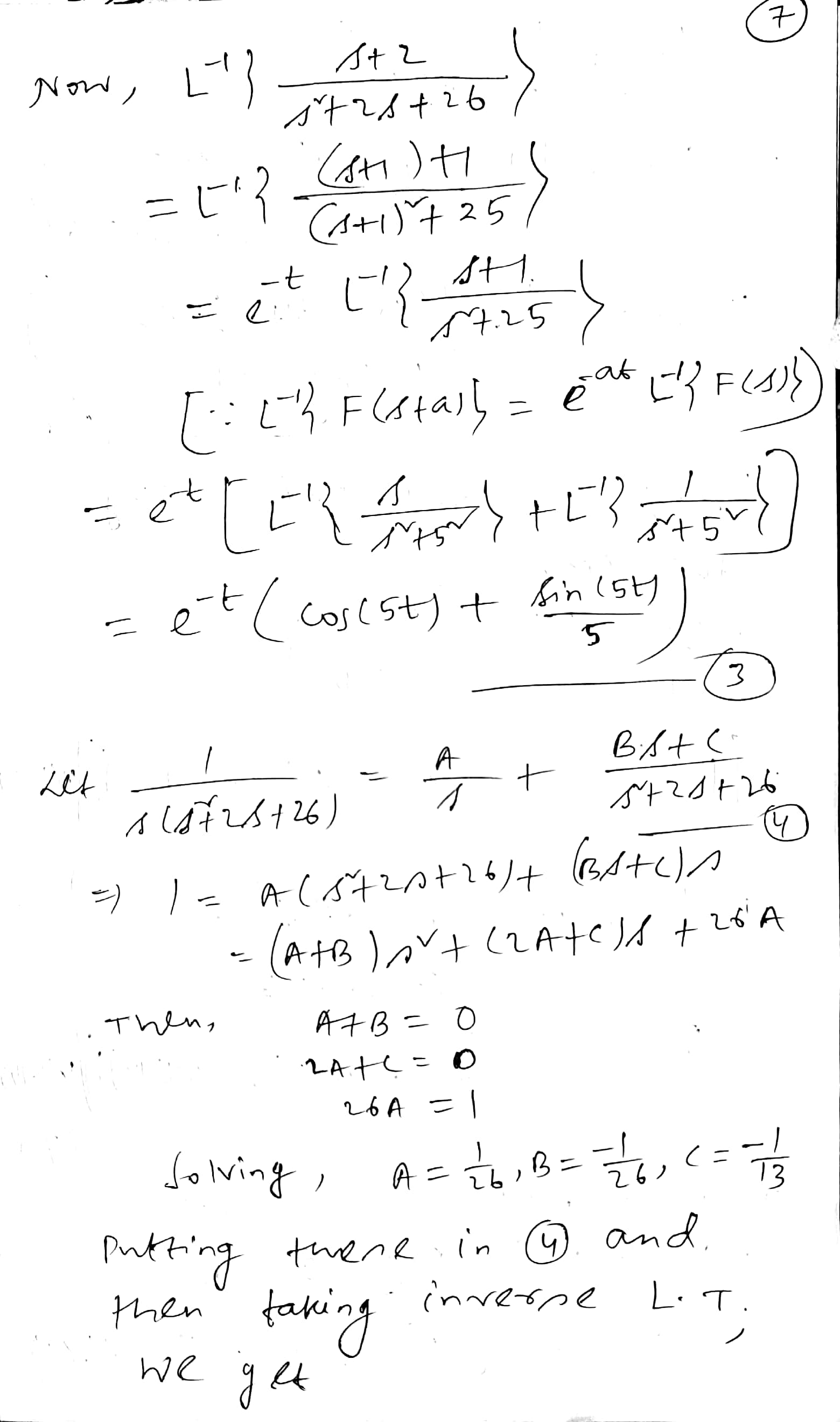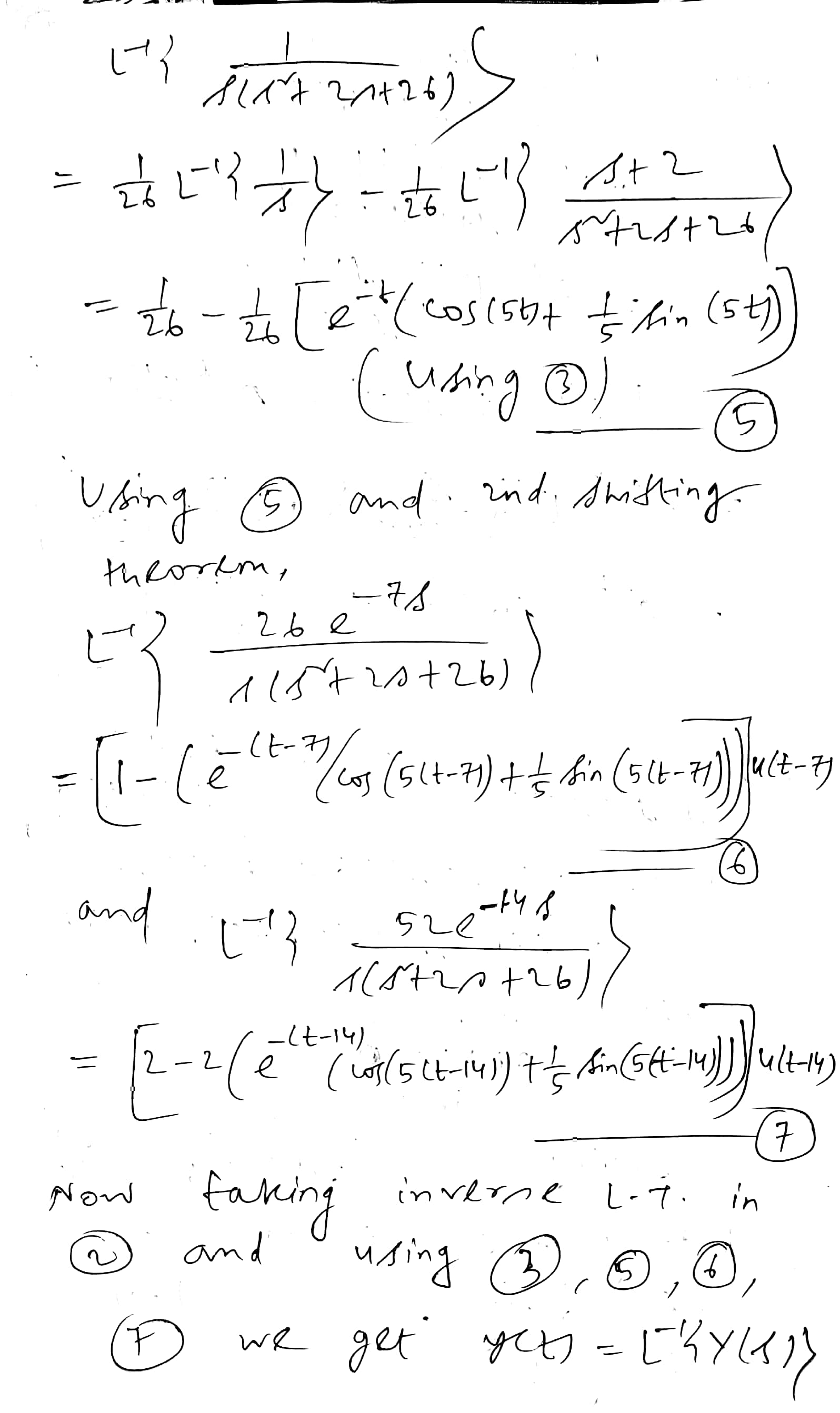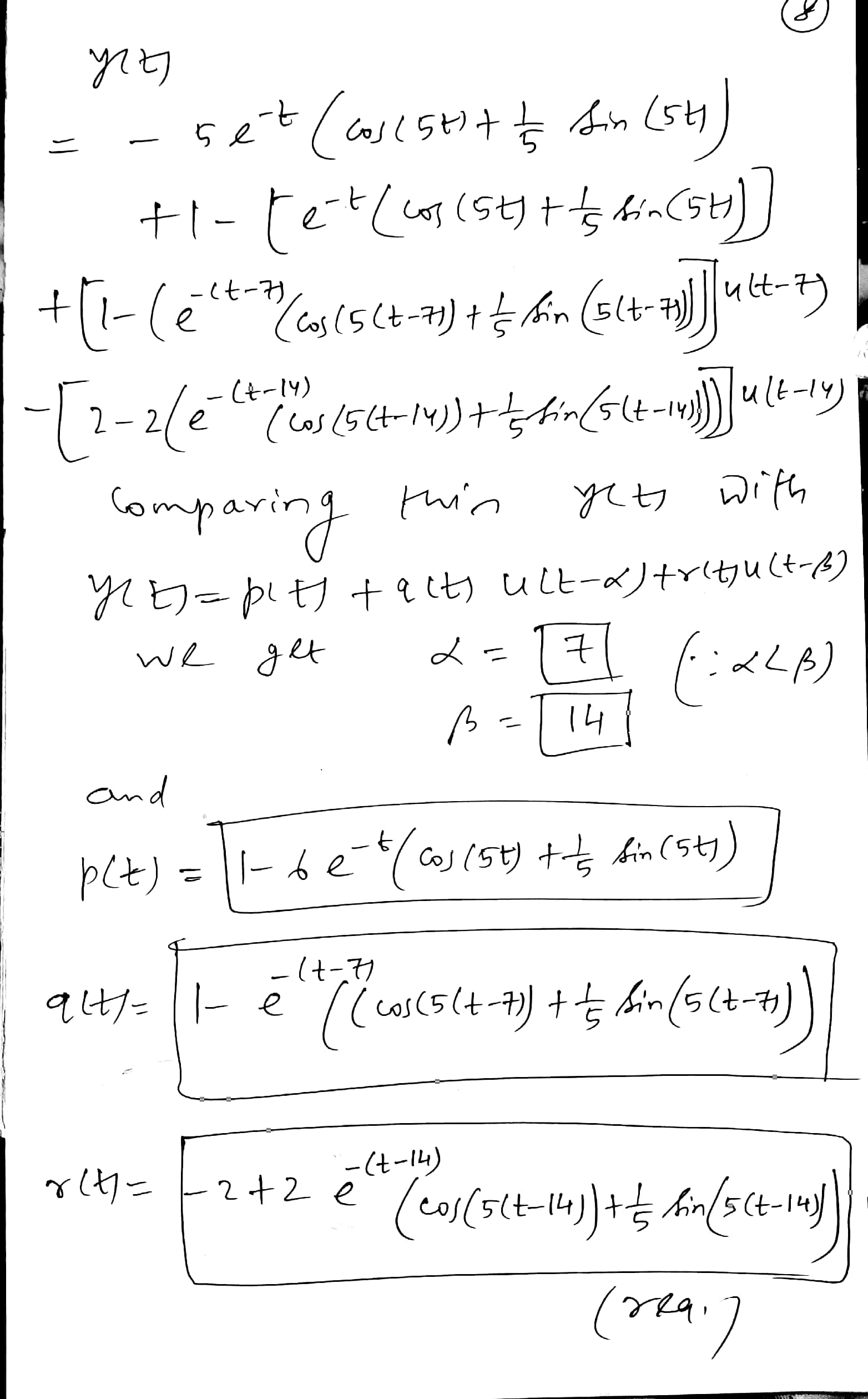##### Add Answer of: Identify p(t), q(t) and r(t). Solve the given initial value problem using the method of Laplace...
Similar Homework Help Questions
• ### Solve the initial value problem below using the method of Laplace transforms. y"' + y' -...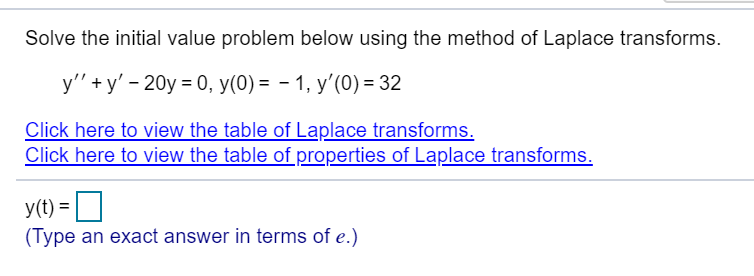Solve the initial value problem below using the method of Laplace transforms. y"' + y' - 20y = 0, y(0) = -1, y'(0) = 32 Click here to view the table of Laplace transforms. Click here to view the table of properties of Laplace transforms. y(t) = (Type an exact answer in terms of e.)

• ### Solve the initial value problem below using the method of Laplace transforms. y" - 2y' -...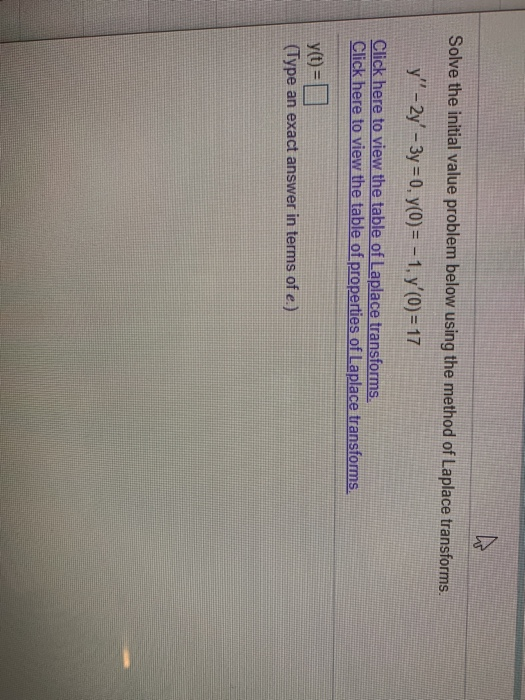Solve the initial value problem below using the method of Laplace transforms. y" - 2y' - 3y = 0, y(0) = -1, y' (O) = 17 Click here to view the table of Laplace transforms. Click here to view the table of properties of Laplace transforms y(t) = 1 (Type an exact answer in terms of e.)

• ### Solve for Y(s), the Laplace transform of the solution y(t) to the initial value problem below....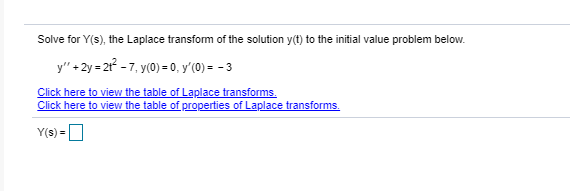Solve for Y(s), the Laplace transform of the solution y(t) to the initial value problem below. y" +2y = 2+2 -7,y(0) = 0, y'(0) = - 3 Click here to view the table of Laplace transforms. Click here to view the table of properties of Laplace transforms. Y(s)-

• ### Solve for Y(s), the Laplace transform of the solution y(t) to the initial value problem below....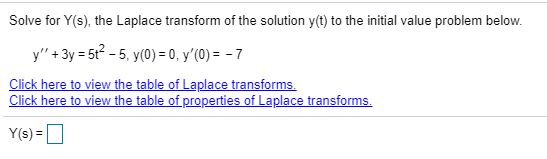Solve for Y(s), the Laplace transform of the solution y(t) to the initial value problem below. y" + 3y = 52 - 5, y(0) = 0, y'(0) = -7 Click here to view the table of Laplace transforms. Click here to view the table of properties of Laplace transforms. Y(s)=

• ### Solve for Y(s), the Laplace transform of the solution y(t) to the initial value problem below....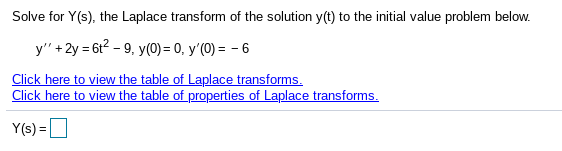Solve for Y(s), the Laplace transform of the solution y(t) to the initial value problem below. y" +2y = 62 -9, y(0) = 0, y'(0) = -6 Click here to view the table of Laplace transforms. Click here to view the table of properties of Laplace transforms. Y(s) =

• ### Solve for Y(s), the Laplace transform of the solution y(t) to the initial value problem below....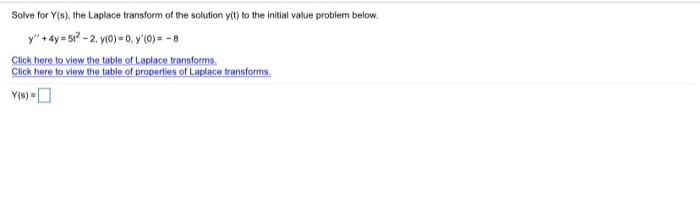Solve for Y(s), the Laplace transform of the solution y(t) to the initial value problem below. y" + 4y = 512 - 2. y(0)=0, 7(0) = -8 Click here to view the table of Laplace transforms Click here to view the table of properties of Laplace transforms. Solve for Y(s), the Laplace transform of the solution y(t) to the initial value problem below. y" + 4y = 5t2 - 2. y(0) = 0, y'(O) = - 8 Click here to...

• ### Solve the initial value problem below using the method of Laplace transforms. 4ty'' - 6ty' +...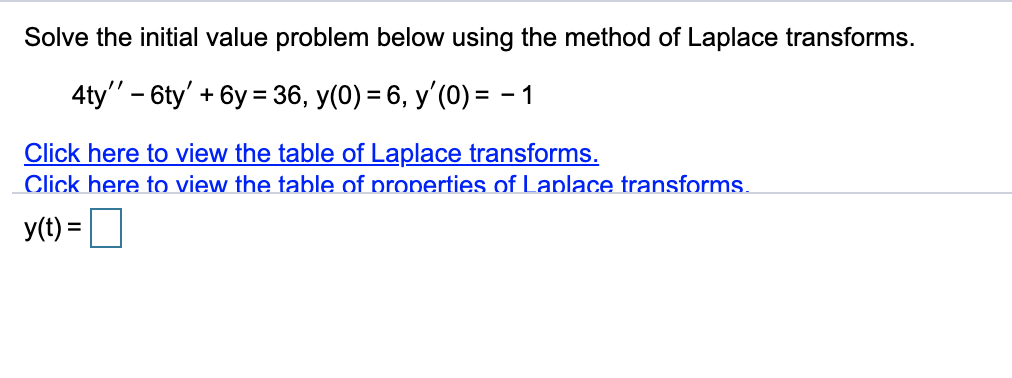Solve the initial value problem below using the method of Laplace transforms. 4ty'' - 6ty' + 6y = 36, y(0) = 6, y'(0) = -1 Click here to view the table of Laplace transforms. Click here to view the table of properties of Laplace transforms. y(t) =

• ### Solve the initial value problem below using the method of Laplace transforms. 2ty" - 5ty' +...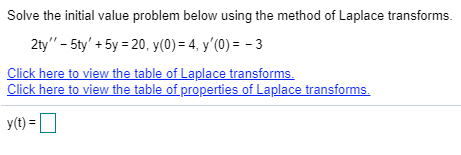Solve the initial value problem below using the method of Laplace transforms. 2ty" - 5ty' + 5y = 20, y(0) = 4, y'0) = -3 Click here to view the table of Laplace transforms. Click here to view the table of properties of Laplace transforms. y(t) =

• ### Solve for Y(s), the Laplace transform of the solution y(t) to the initial value problem below....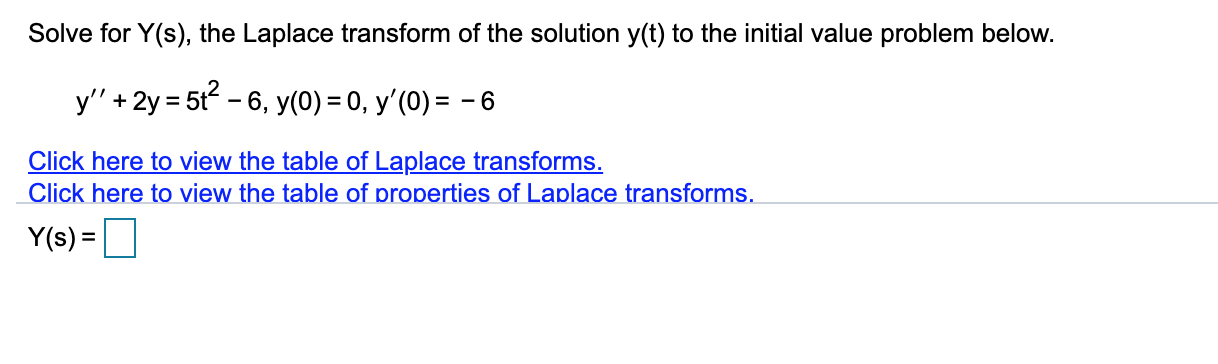Solve for Y(s), the Laplace transform of the solution y(t) to the initial value problem below. y'' + 2y = 562 - 6, y(0) = 0, y'(O) = - 6 Click here to view the table of Laplace transforms. Click here to view the table of properties of Laplace transforms. Y(s) = 1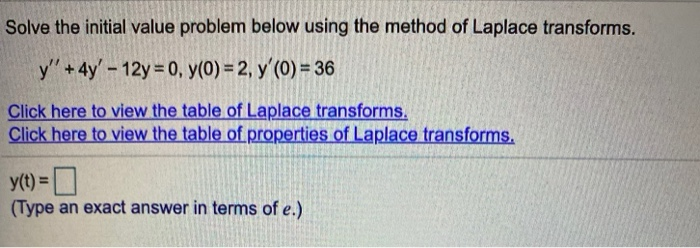please please please answer all! its very appreciated! Solve the initial value problem below using the method of Laplace transforms. y'' + 4y' - 12y = 0, y(0) = 2, y' (O) = 36 Click here to view the table of Laplace transforms. Click here to view the table of properties of Laplace transforms. y(t) = 0 (Type an exact answer in terms of e.) Solve the initial value problem below using the method of Laplace transforms. y'' - 8y'...

Free Homework App